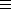## CGAL::Polyhedron_3<Traits>::Vertex

### Definition

A vertex optionally stores a point and a reference to an incident halfedge that points to the vertex. Type tags indicate whether these member functions are supported. Figuredepicts the relationship between a halfedge and its incident halfedges, vertices, and facets. The circulator is assignable to the Halfedge_handle. The circulator is bidirectional if the halfedge provided to the polyhedron with the Items template argument provides a member function prev(), otherwise it is of the forward category.

#include <CGAL/Polyhedron_3.h>

### Types

 Polyhedron_3::Vertex::Halfedge type of incident halfedges. Polyhedron_3::Vertex::Facet type of incident facets. Polyhedron_3::Vertex::Point_3 point type stored in vertices. Polyhedron_3::Vertex::Vertex_handle handle to vertex. Polyhedron_3::Vertex::Halfedge_handle handle to halfedge. Polyhedron_3::Vertex::Facet_handle handle to facet. Polyhedron_3::Vertex::Halfedge_around_vertex_circulator circulator of halfedges around a vertex. Polyhedron_3::Vertex::Vertex_const_handle Polyhedron_3::Vertex::Halfedge_const_handle Polyhedron_3::Vertex::Facet_const_handle Polyhedron_3::Vertex::Halfedge_around_vertex_const_circulator Polyhedron_3::Vertex::Supports_vertex_halfedgeCGAL::Tag_true or CGAL::Tag_false. Polyhedron_3::Vertex::Supports_vertex_pointCGAL::Tag_true or CGAL::Tag_false.

### Creation

 Polyhedron_3::Vertex v; default constructor. Polyhedron_3::Vertex v ( Point p); vertex initialized with a point.

### Operations available if Supports_vertex_pointCGAL::Tag_true

 Point_3& v.point () const Point_3& v.point () const the point.

### Operations available if Supports_vertex_halfedgeCGAL::Tag_true

 Halfedge_handle v.halfedge () Halfedge_const_handle v.halfedge () const an incident halfedge that points to v. Halfedge_around_vertex_circulator v.vertex_begin () Halfedge_around_vertex_const_circulator v.vertex_begin () const circulator of halfedges around the vertex (clockwise). void v.set_halfedge ( Halfedge_handle h) sets incident halfedge to h. Precondition: h is incident, i.e., h->vertex() == v. std::size_t v.vertex_degree () const the degree of the vertex, i.e., number of edges emanating from this vertex. bool v.is_bivalent () const returns true if the vertex has exactly two incident edges. bool v.is_trivalent () const returns true if the vertex has exactly three incident edges.